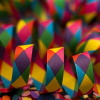You may also likeRationals Between...

What fractions can you find between the square roots of 65 and 67?Repetitiously

Can you express every recurring decimal as a fraction?Equal Equilateral Triangles

Can you make a regular hexagon from yellow triangles the same size as a regular hexagon made from green triangles ?

The Square Hole

Why do this problem?

The bisected equilateral triangle ($30-60-90$) is an important shape for students to become familiar with. Each of the $4$ rectangles is made from $2$ equilateral triangles and $2$ isosceles triangles. Together one of each of those two, makes the $30-60$ right-angled triangle.

This problem forces consideration of the side lengths in surd form, and provides an opportunity to become familiar with manipulating surd forms.

The switch from one quantity as the given unit to another, emphasises that the choice of unit is a choice for the problem solver and can be considered so as to make the relationships within a problem as clear as possible.

Possible approach

Preceding these questions with 'playtime' using cut out triangles to form patterns may be a very useful preliminary for many students.

Include shapes that have holes, and suggest/invite challenges with respect to that 'hole'.

And maybe include the challenge to lose the 'hole' but keep the square.

Key questions

• What is the area of the yellow equilateral triangle in terms of its side length ?

• What is the relationship between the area of the yellow triangle and the area of the purple triangle ? [they are equal]

Possible extension

Impossible Triangles? is a good next step, along with the extension suggestions in Equal Equilateral Triangles

Possible support

There are a number of activities which can provide valuable auxiliary experiences for students working on this problem :

Drawing first the equilateral triangle using only a straight edge and compasses, and then creating the isosceles triangle, likewise, will give a strong sense for the symmetry of each triangle and the relationship between them.

Some children 'play' a long time with cut out triangles arranged on a table, motivated by the aesthetic appeal of emerging pattern possibilities, this is excellent grounding for other mathematical ideas to be built on.